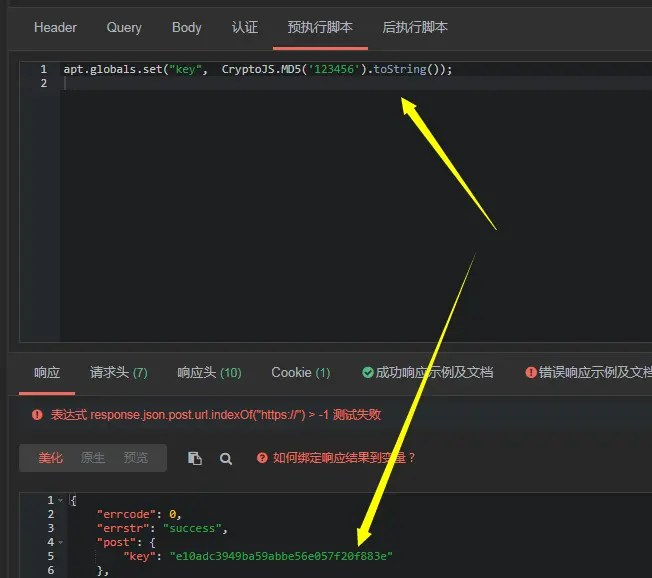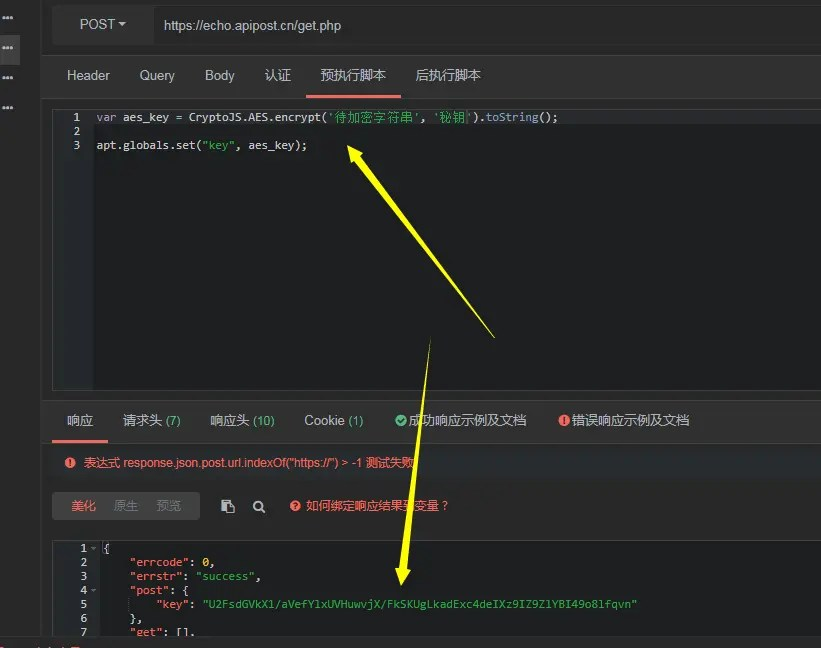# Methods and examples of MD5/SHA256/BASE64/AES encryption and decryption using CryptoJS in JS

Posted Jun 5, 20203 min read

CryptoJS(crypto.js) provides various encryption algorithms for JavaScript, because it is slightly more complicated to use. So this article mainly focuses on CryptoJS MD5/SHA256/BASE64/AES encryption and decryption methods and examples.

You can download CryptoJS( https://github.com/brix/crypto-js ) Go to your local area, create a new webpage and debug through console.log(), you can use an interface management tool ApiPost( https://www.apipost.cn/ ) For debugging.

ApiPost is a tool with functions similar to Postman, but it incorporates the document sharing function, which is very convenient to use. It has built-in CryptoJS support, so I use it as a debugging tool.

## MD5 encryption

``CryptoJS.MD5('String to be encrypted').toString()``## SHA256 encryption

``CryptoJS.SHA256('String to be encrypted').toString()``## base64 encryption

``CryptoJS.enc.Base64.stringify(CryptoJS.enc.Utf8.parse('String to be encrypted'))``## base64 decryption

``CryptoJS.enc.Base64.parse("String to be decrypted").toString(CryptoJS.enc.Utf8)``## AES simple encryption

``CryptoJS.AES.encrypt('String to be encrypted','Key').toString()``## AES simple decryption

``CryptoJS.AES.decrypt('String to be decrypted','Key').toString(CryptoJS.enc.Utf8)``## Custom AES encryption and decryption functions

The above examples are 2 simple aes encryption and decryption schemes. In most cases, we need to customize more parameters for aes encryption and decryption, such as encryption mode and padding.

``````Const key = CryptoJS.enc.Utf8.parse("secret key"); //16-digit hexadecimal number as the key
const iv = CryptoJS.enc.Utf8.parse('Offset'); //16-bit hexadecimal number as the key offset

//Decryption method
function Decrypt(word) {
let encryptedHexStr = CryptoJS.enc.Hex.parse(word);
let srcs = CryptoJS.enc.Base64.stringify(encryptedHexStr);
let decryptedStr = decrypt.toString(CryptoJS.enc.Utf8);
return decryptedStr.toString();
}

//Encryption method
function Encrypt(word) {
let srcs = CryptoJS.enc.Utf8.parse(word);